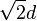# Difference between revisions of "Gain score"

## Definition

The term gain score is used to refer to the difference between the scores (typically, raw scores) on a posttest and pretest that are either identical or have scores with the same meaning. Explicitly:

Gain score = (Raw score on posttest) - (Raw score on pretest)

The gain score can be measured either for an individual (who takes both the pretest and the posttest) or for a group of individuals (each of whom individually takes both the pretest and the posttest). The latter is termed the average gain score.

## Related notions

Term Meaning
average gain score for a group of individuals (typically, the group is subject to some instruction, with the pretest just before the instruction and the posttest just after the instruction) average of their respective gain scores; equivalently, difference between average posttest score and average pretest score (note that taking the difference and taking the average are commuting operations).
normalized gain score for an individual the quotient of the gain score by the maximum possible gain score. If scores are measured out of 1, it is given as (gain score)/(1 - raw score on pretest).
average normalized gain score for a group of individuals the average of the normalized gain scores for a group of individuals
normalized average gain score for a group of individuals we normalize after computing the average scores. It is given as (average gain score)/(1 - average raw score on pretest).

## Reliability

One of the main concerns with measuring gain scores is whether they are sufficiently reliable. The reliability here is relative to the natural measurement error in the testing instrument due to random variation or other factors. For instance, suppose that a student's expected score on a test follows a distribution similar to a normal distribution (albeit, with appropriate censoring) centered at the student's "true score." The actual score on the test is a random variable from this distribution.

What we would like to measure is the "true pretest score" and the "true posttest score" and then take the difference. In reality, we sample from the distributions for the pretest and posttest scores, and take the difference. The extent to which the errors augment or cancel each other depends on how they are correlated.

### Case of uncorrelated measurement errors

In case the measurement errors on the two tests are uncorrelated (since it's the same test, that's basically saying that the measurement error of two different administrations of the same test are uncorrelated), then the variances of the distributions add. If both measurements have a standard deviation of$d$, the standard deviation of the difference is$\sqrt{2}d$.

The means, however, subtract. Therefore, the standard deviation-to-mean ratio could be quite high. For instance, if the means are 0.2 and 0.24 respectively for the pretest and posttest, and the standard deviation is 0.03, then the standard deviation-to-mean ratio is 0.03/0.04 = 0.75, which is very high. In particular, with such a high standard deviation-to-mean ratio, we may not even be sure of the sign of our measurements

### Case of correlated measurement errors

If the measurement errors on the pretest and posttest are positively correlated (for instance, a student may be unaware of an English word that hampers his ability to do a particular test item on both pretest and posttest), then the measurement error for the gain score would be correspondingly less. In the perfect situation where both measurement errors are perfectly correlated, the gain score is completely reliable.